# Scouts 4

4/7 of the students in a school are boys. If 3/8 of the boys are scouts, how many scouts are there in a school of 1878 students?

Correct result:

s =  402.4286

#### Solution: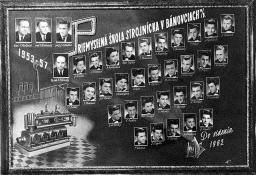We would be pleased if you find an error in the word problem, spelling mistakes, or inaccuracies and send it to us. Thank you!Tips to related online calculators
Need help calculate sum, simplify or multiply fractions? Try our fraction calculator.

## Next similar math problems:

• Summerjobs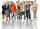The agency give summerjobs for 2352 students in 2018. The eighth was high-school students, the rest was undergraduates. How many undergraduates works via agency in 2018?
• One third 2One third of all students in class live in a house. If here are 42 students in a class, how many of them live in house?
• School trip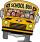School trip cost 247.2 Eur for one class (24 students). How much would cost a trip for two classes? (both classes together have 53 students)
• Report 2A School reports students to teacher ratio of 6:1. If there are 45 teachers in the School, how many students are there?
• Four-member teamThere are 14 girls and 11 boys in the class. How many ways can a four-member team be chosen so that there are exactly two boys in it?
• Students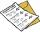The class has 22 students. 2 of which has assesement of 2. Count of assesement of 1 is three times more than count of students with assesement of 3. How many students has assesement of 1 and how many students has assesement of 1.
• Students at cinema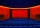All pupils from the school went to the cinema for a film performance. The cinema has 400 seats, 2 rows of 20 seats remain unoccupied. 10 places were occupied by teachers. How many students were there?
• Class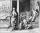When Pythagoras asked how many students attend the school, said: "Half of the students studying mathematics, 1/4 music, seventh silent and there are three girls at school." How many students had Pythagoras at school?
• Math test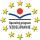In class 6.A are 26 students and the teacher had managed to give tests to 13 students. 13 testes he gave in 6.5 min. How many pupils still haven't test? How long will it take techer to give tests to 43 students?
• Pairs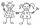From the five girls and four boys teachers have to choose one pair of boy and girl. A) How many such pairs of (M + F)? B) How many pairs where only boys (M + M)? C) How many are all possible pairs?
• ClassroomOne-eighth of 9th class was interested in studying at a grammar school, at a business academy one sixth, at secondary vocational schools quarter, to SOU one third and the remaining three students were interested in the school of art direction. How many st
• Fractions 3Calculate 1/9 of 27:
• Pupils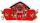Two classes to collect money. Boys are four sevenths pupils. In time did not pay a quarter of the boys and a sixth of girls, which together mean 12 sinners. How many pupils attend this two classes ?
• The classroom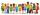In the seventh class the number of boys and girls are at a ratio of 4:5. Boys are 16. How many total students are in the classroom?
• CompareCompare fractions ?. Which fraction of the lower?
• NumbersDetermine the number of all positive integers less than 4183444 if each is divisible by 29, 7, 17. What is its sum?
• Foot in busIt was 102 people on the bus. 28 girls had two dogs. A 11 girls had one dog. At the next stop seceded 5 dogs (even with their owners). They got two boys together with three dogs. The bus drove one driver. How many foot were in bus?